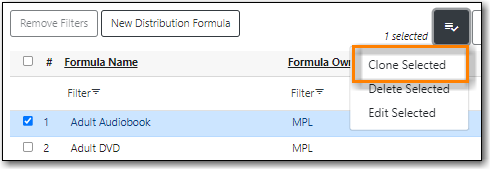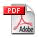## Distribution Formulas

Creating a Distribution Formula
Editing a Distribution Formula
Cloning a Distribution Formula

Distribution formulas are intented for use in multi-branch systems to specify the number of copies that should be distributed to specific branches.

The formulas are also used for all libraries as line item templates with the batch updater on purchase orders. Formulas can be created and reused as needed.

### Creating a Distribution Formula

2. Click New Distribution Formula.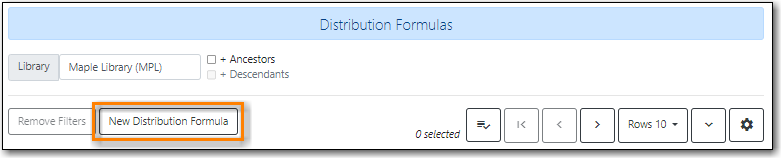3. Enter a formula name. There is no limit to the number of characters that can be entered in this field.
4. Choose a formula owner. The formula owner indicates the organizational units whose staff can use this formula.
5. Create your distribution formula/line item template using a combination of the following fields:

• Owning Library
• Shelving Location
• Fund
• Circ Modifier
• Collection Code
6. In the Item Count field, enter the number of copies that should be added to the branch.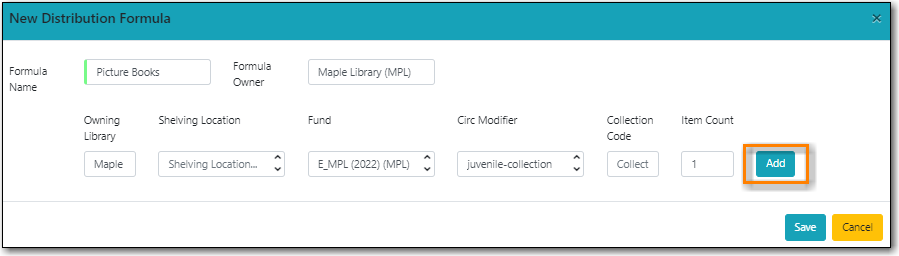8. Multi-branch libraries can add lines for as many branches as you require.
9. Click Save.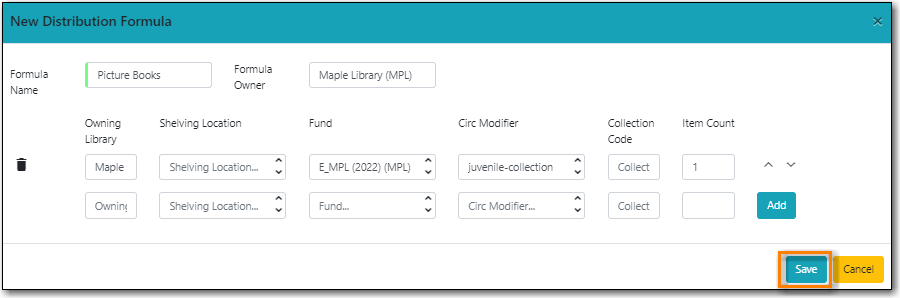### Editing a Distribution Formula

2. Select the formula you want to edit and from the Actions menu choose Edit Selected.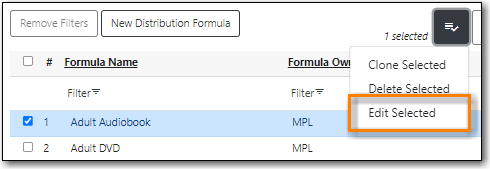3. On the pop up you can add a new entry, edit an existing entry, or delete an existing entry using the trash can icon.
4. Use the arrows to re-order the entries.

1. When using a distribution formula the system will start at the top of the list and work down the list until it runs out of items. For example, if you are ordering five copies of a title and you apply a distribution formula with entries for seven branches (1 item each), only the first five branches will receive items.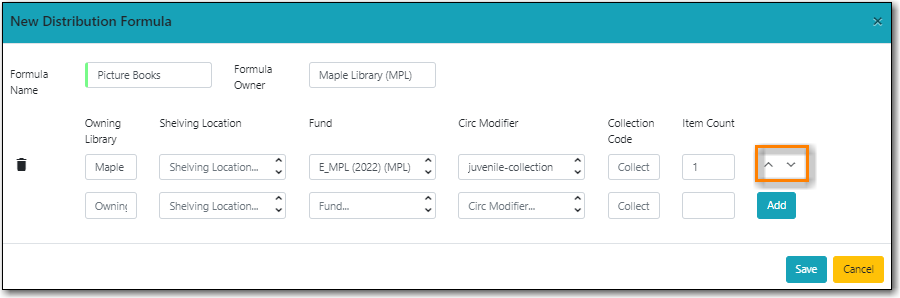### Cloning a Distribution Formula